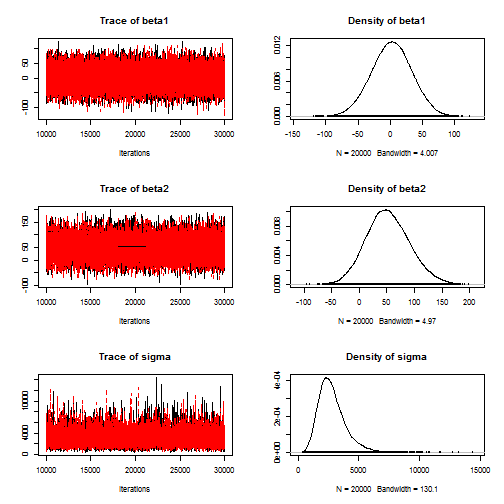# Using JAGS for MCMC sampling

### Chapter 3.3: Introduction to JAGS

In this example, we use JAGS to conduct simple linear regression. Before executing this code, but sure to install JAGS and the R package rjags.

The response is the mass of a T. Rex and the covariate is the age. The model is $mass_i\sim\mbox{Normal}(\beta_1+\beta_2age_i,\sigma^2).$ The priors are $$\beta_1,\beta_2\sim\mbox{Normal}(0,1000)$$ and $$\sigma^2\sim\mbox{InvGamma}(0.1,0.1)$$.

## Load T-Rex data

 library(rjags)

mass <- c(29.9, 1761, 1807, 2984, 3230, 5040, 5654)
age  <- c(2, 15, 14, 16, 18, 22, 28)
n    <- length(age)

# JAGS require all the data to be packaged as a list
data <- list(mass=mass,age=age,n=n)


## (1) Define the model as a string

 model_string <- textConnection("model{

# Likelihood (dnorm uses a precision, not variance)
for(i in 1:n){
mass[i] ~ dnorm(beta1 + beta2*age[i],tau) #tau = 1/sigma^2
}

# Priors
tau   ~  dgamma(0.1, 0.1)
sigma <- 1/sqrt(tau)
beta1 ~  dnorm(0, 0.001)
beta2 ~  dnorm(0, 0.001)

}")


## (2) Load the data and compile the MCMC code

 inits <- list(beta1=rnorm(1),beta2=rnorm(1),tau=10)
model <- jags.model(model_string,data = data, inits=inits, n.chains=2,quiet=TRUE)


## (3) Burn-in for 10000 samples

 update(model, 10000, progress.bar="none")


## (4) Generate 20000 post-burn-in samples and retain the parameters named in params

 params  <- c("beta1","beta2","sigma")
samples <- coda.samples(model,
variable.names=params,
n.iter=20000, progress.bar="none")


## (5) Summarize the output

 summary(samples)

##
## Iterations = 10001:30000
## Thinning interval = 1
## Number of chains = 2
## Sample size per chain = 20000
##
## 1. Empirical mean and standard deviation for each variable,
##    plus standard error of the mean:
##
##           Mean      SD Naive SE Time-series SE
## beta1    2.477   31.47   0.1573         0.1573
## beta2   51.979   39.04   0.1952         0.3849
## sigma 2809.370 1169.48   5.8474        10.0124
##
## 2. Quantiles for each variable:
##
##          2.5%     25%      50%     75%   97.5%
## beta1  -59.55  -18.58    2.723   23.68   63.58
## beta2  -20.88   25.25   50.475   77.65  132.84
## sigma 1095.13 2015.22 2620.528 3384.47 5647.02

 plot(samples)